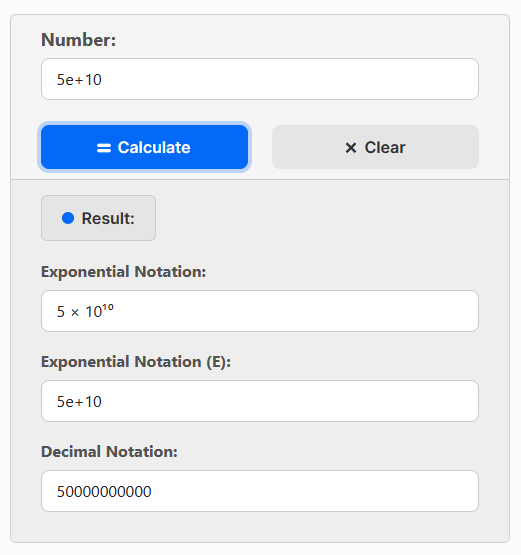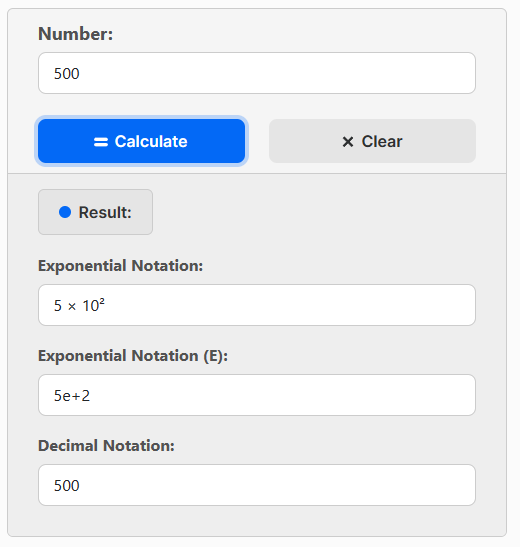# Exponential Notation Calculator

Convert any number to exponential notation, or convert exponential notation to decimal number.

Result:

Exponential notation is a concise way of representing very large or very small numbers using powers of 10. It is commonly used in scientific and mathematical calculations to simplify the representation of numbers.

## Calculator Use

To use the calculator, simply input the numbers in decimal or exponential notation. The calculator will then display the result in exponential notation or in standard decimal form, depending on your preference.

For example:## Converting Decimals to Exponentials

To convert decimals to exponentials, determine the coefficient and the exponent.

1. To get the coefficient, move the decimal point to the left until there is only one non-zero digit to the left of the decimal point. Count the number of places you moved the decimal point.
2. The exponent represents the number of places the decimal point was moved. It is equal to the number of places you moved the decimal point.
3. Write the coefficient followed by the letter "x" and then write 10 raised to the power of the exponent.
Example: Convert 5,200,000 to exponential notation:

1. The coefficient is 5.2 because we move the decimal point six places to the left.

2. As we moved it six places, the exponent is 6.

3. The number 5,200,000 in exponential notation is 5.2 x 106 or 5.2e+6

## Converting Exponentials to Decimals

To convert a number from exponential notation to decimal form, multiply the coefficient by 10 raised to the power of the exponent.

Example: Convert 3.5 x 103 (or 3.5e+3) to decimal notation:

Multiply 3.5 by 10 three times:

3.5 * 10 * 10 * 10 = 3500

The result is 3500.

## Examples

Number Exponential Notation Exponential Notation (E)
200 2 × 10² 2e+2
250 2.5 × 10² 2.5e+2
5000 5 × 10³ 5e+3
70000 7 × 10⁴ 7e+4
8543 8.543 × 10³ 8.543e+3
0.0004 4 × 10⁻⁴ 4e-4
Math Calculators
PureTables.com
Have an idea for a tool or calculator that would make your life easier? Contact us, so we could try to create and provide it.